## COMP 256 Programming Assignment 1

Photometric stereo. (assignment)

This homework got some press coverage on the Science & Technology Department of the French Embassy in the United Kingdom web site, in a press review (see page 43) about security at the 2012 Olympic Games. A similar article has been published in Biometrie-Online. It also got listed on The Graphics and Media Lab page.

### Algorithm

First I implemented the algorithm described on page 86 of Forsyth and Ponce. I used a MatLab script to generate a set of images as a test problem. From images such as the ones below, I was able to reconstruct the albedo shown in the rightmost image.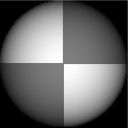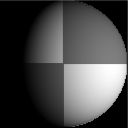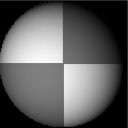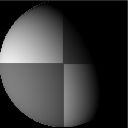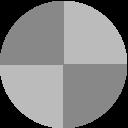The computed derivatives of the function are shown below: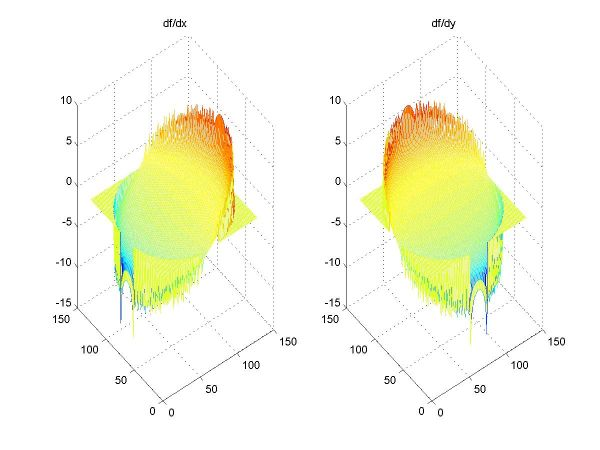The integration algorithm proposed in the book did not yield satisfactory results: while it seemed to do an average job on sharp edges, smooth surfaces were noisy. I chose to use an implementation of the multigrid 2D integration algorithm from "Numerical Recipes in C++". It did a much better job on the test case, as seen below. Basically, the multigrid algorithm iteratively solves a global minimization problem, while the simple integration is single-pass, and more sensitive to the propagation of local errors.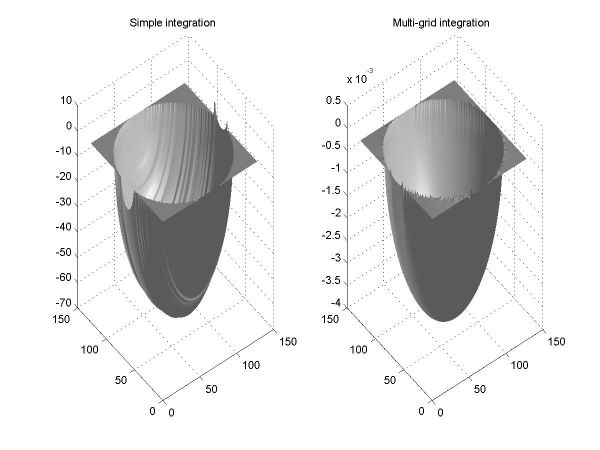Simply integrating yielded satisfactory results for the test case, as shown below (left: the recovered surface; right: the surface, textured with the albedo).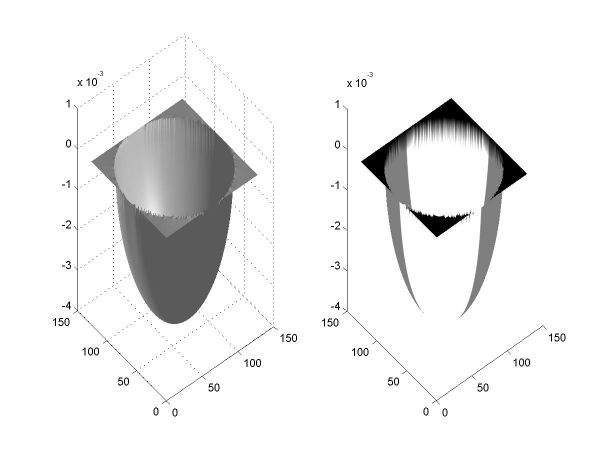When run on the data from the face database (yaleB01_P00), the algorithm above did not produce good results. After applying several preprocessing steps described later in this report, the recovered albedo is the one shown below.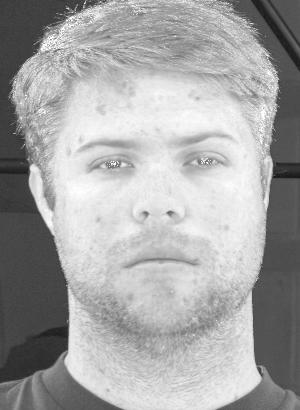The images below show the computed derivatives (clamped) and the comparison between simple and multigrid integration.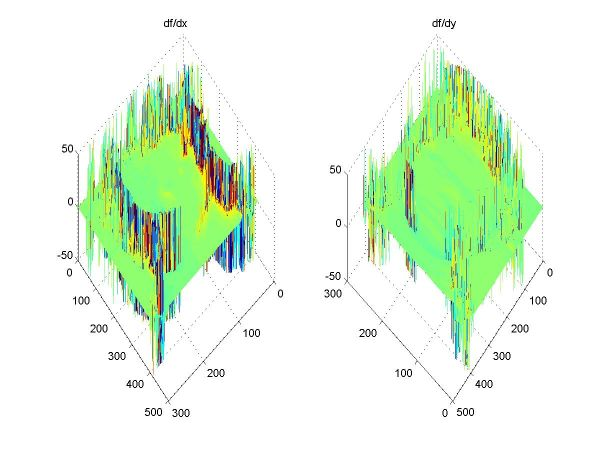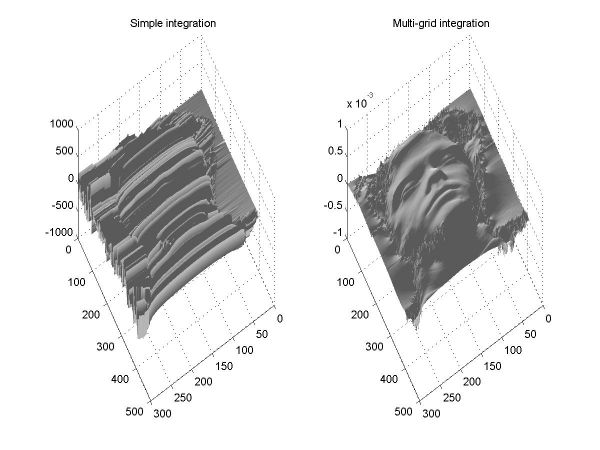### Heuristics

I applied several heuristics to try to improve the results.

Subtracting the ambient image form the images in the dataset yielded a few negative values. I clamped them to zero. At first I did not notice the bug in the image loading routine, and I was subtracting the wrong ambient image. This yielded entire areas of negative values, for which I tried several heuristics (clamping to zero, leaving them negative, negating them, and replacing them with white noise). Since subtracting the correct ambient image yields very few negatives, I chose not to include the images in this report, as the heuristics have very little effect on the results.

The values of the derivatives had a few large spikes due to numerical errors. I tried several ways to filter them out, using Gaussians and sanity checks of the cross derivatives, but the single most efficient method was also the simplest: clamping the derivatives to the narrow domain [-10..10] provided satisfactory results. The other methods only provided marginal improvements in the visual appearance of the result. This is mainly due to the fact that the multigrid integration algorithm finds the closest approximation of the function by iteratively solving a minimization problem, which by itself alleviates this type of problems. The initial results after clamping are shown below.

 The initial results after clamping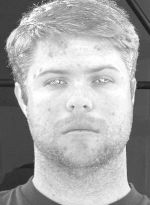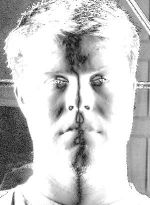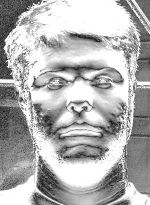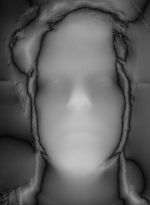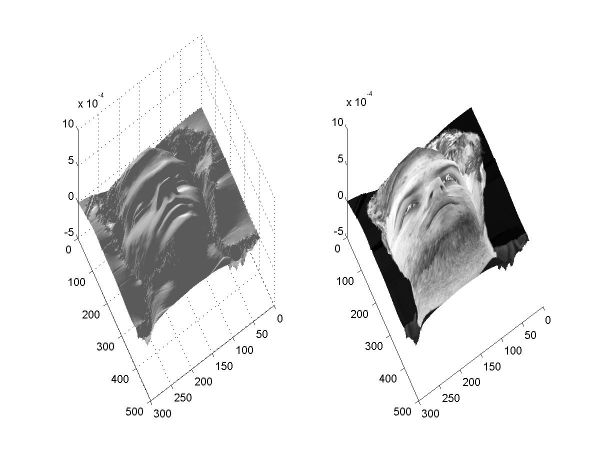albedo df/dx df/dy height map result

I wanted to find a way to eliminate specularities. One method would be to manually select the appropriate images. An automatic, pixel-by-pixel approach should cancel out the equations corresponding to the images where a particular pixel is specular from the linear system used to solve for the surface normal at that pixel. To do so, I first store the values for points that are white, yet non-specular (i.e., white in the majority of the images), then eliminate all white points by setting them to zero, then restore the values for the non-specular points. For this particular dataset, there were no such non-specular points for my chosen thresholds (how many images constitute the "majority" and how bright "white" actually is), so I comented out the section of the code that performed the restoration and simply set the white points to zero. I considered values higher than 230 on a scale of 0 to 255 to be "white". The result is a smoother reconstruction in the zones with specularities, such as the eyes and the tip of the nose.

 The results after eliminating the specularities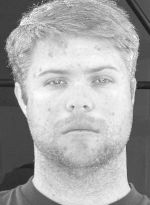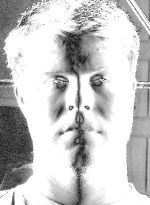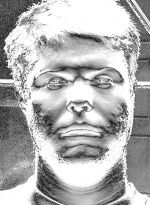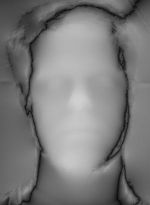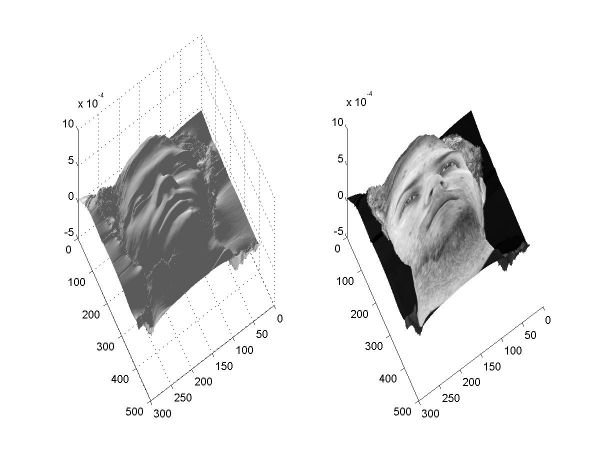albedo df/dx df/dy height map result

To smooth the output further, I tried blurring the input images by convolving them with a Gaussian function. This results in a blurred albedo and a smoother height map, as shown below.

 The results of blurring the source images with a Gaussian of size 4 and sigma 2.5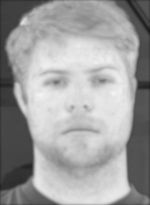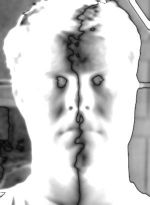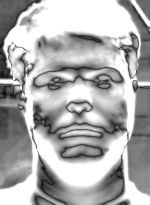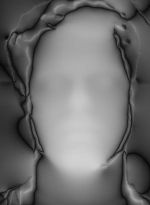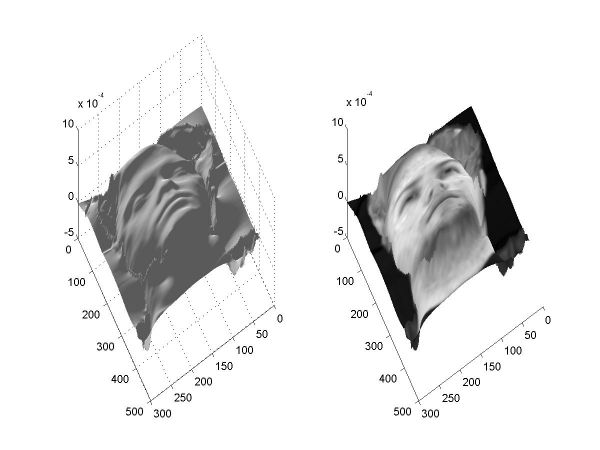albedo df/dx df/dy height map result

As expected, blurring the derivatives instead results in an even smoother height map, while the albedo remains sharp.

 The results of blurring the derivatives with a Gaussian of size 4 and sigma 2.5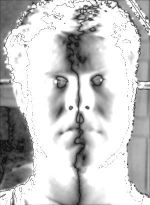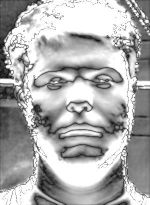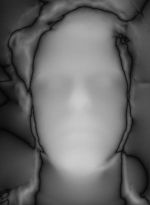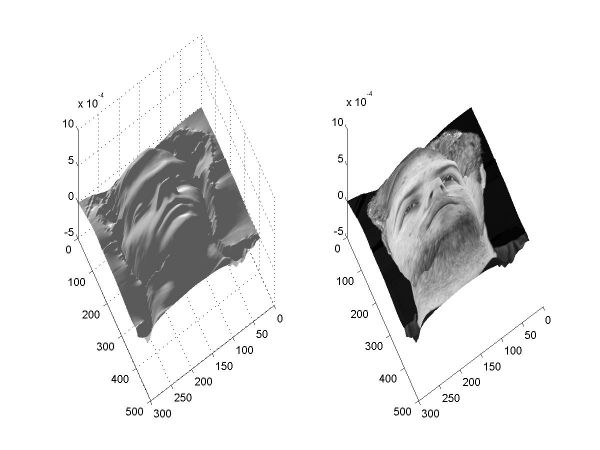albedo df/dx df/dy height map result

Note: Images 2, 3 and 4 in each row above contain both positive and negative values. The images show the absolute value at each pixel. Thus, a brighter color signifies a value further away from 0, while a darker color signifies a value closer to 0. This is the default convention used for color mapping of high dynamic range images in HDR Shop, which I used to save images 1 through 4 in each row above as JPEGs.

To execute the examples first download all the files and unzip mg.exe. Then, run assgn1.m or assgn1toy.m; wait until processing is done (MatLab stops printing); run the executable mg.exe to perform the integration; and press a key in MatLab to visualize the results. process.m computes the albedo and the partial derivatives given a set of images (without smoothing the derivatives, the code is comented out), and finalize.m shows the results. The executable mg.exe takes as input two floating-point images containing the values of the partial derivatives in the .pfm format and outputs a floating point image containing the result of the integration.